# Existence of a unique positive solution for a singular fractional boundary value problem

### Abstractcarpathian_2018_34_1_57_64_abstract

In the present work, we discuss the existence of a unique positive solution of a boundary value problem for a nonlinear fractional order equation with singularity. Precisely, order of equation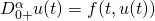belongs to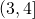and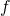has a singularity at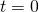and as a boundary conditions we use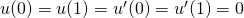. Using a fixed point theorem, we prove the existence of unique positive solution of the considered problem.